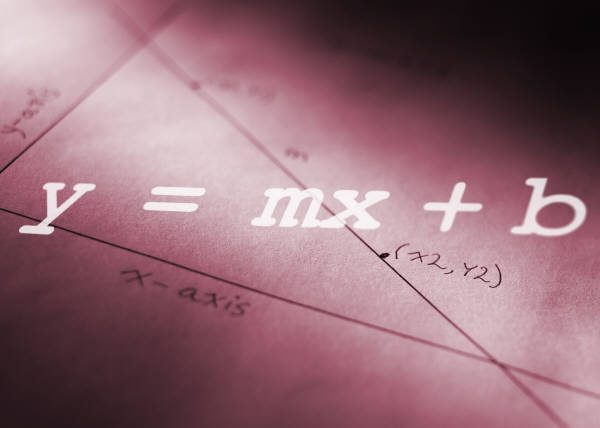## Algebra I

Algebra I is considered to be the 1st course in a series of 4-5 math courses that a student will take over a their high school career.  Much of a student's future math success will be based upon core skills learned in Algebra I.The following concepts will be taught in the
Algebra I Seminar:

1. Multi-step algebra equations
2. Graphing linear equations
3. Calculating rate of change and slope
4. Write the equation of a Line given two points
5. Solve systems of equations both graphically and algebraically

Core Math Seminars
Ashburn, VA 20147
ph: 703.201.9995
CoreMathTutoring@gmail.com